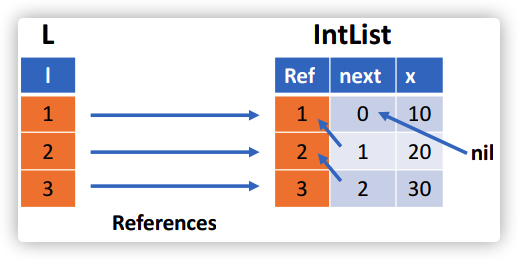# Datalog语法

Datalog主要用于描述关系，是一种声明式逻辑编程语言，目前最常见的一种实现Souffle：https://souffle-lang.github.io/

## 谓词（Predicate/Relation）

• 本质上，谓词是一个数据表;
• 一个Fact代表一个特定的元组（也就是数据表的某一行）属于某一个Relation（也就是某一个表），即它代表一个谓词对于特定的值组合为真；

personage
C0m3ct3
P1n93r18

## 原子（Atom）

• 主要分为两种原子：关系型原子（Relational atom）和算术型原子（arithmetic atom）；
• 例如 `Age(”C0m3ct”,3)` 就是一个关系型原子，而 `age>18` 则是一个算术型原子；

## 规则（Rule）

• Rule是逻辑表达式推理的一种方式；
• Rule还用于指定如何推断事实；
• Rule的格式为： `H``:- B1,B2,…,Bn.` ，注意最后有一个点；这个Rule表明，如果H为真，则 `B1,B2,…,Bn` 也必须为真；

## 逻辑（Logic）

• 逗号表示逻辑与；
• 封号表示逻辑或；

 ``````1 2 `````` ``````Audit(person) :- Age(person,age),age > 18. Audit(person) :- Age(person,age),age = 18. ``````

# 安装Souffle

 ``````1 `````` ``````brew install --HEAD souffle-lang/souffle/souffle ``````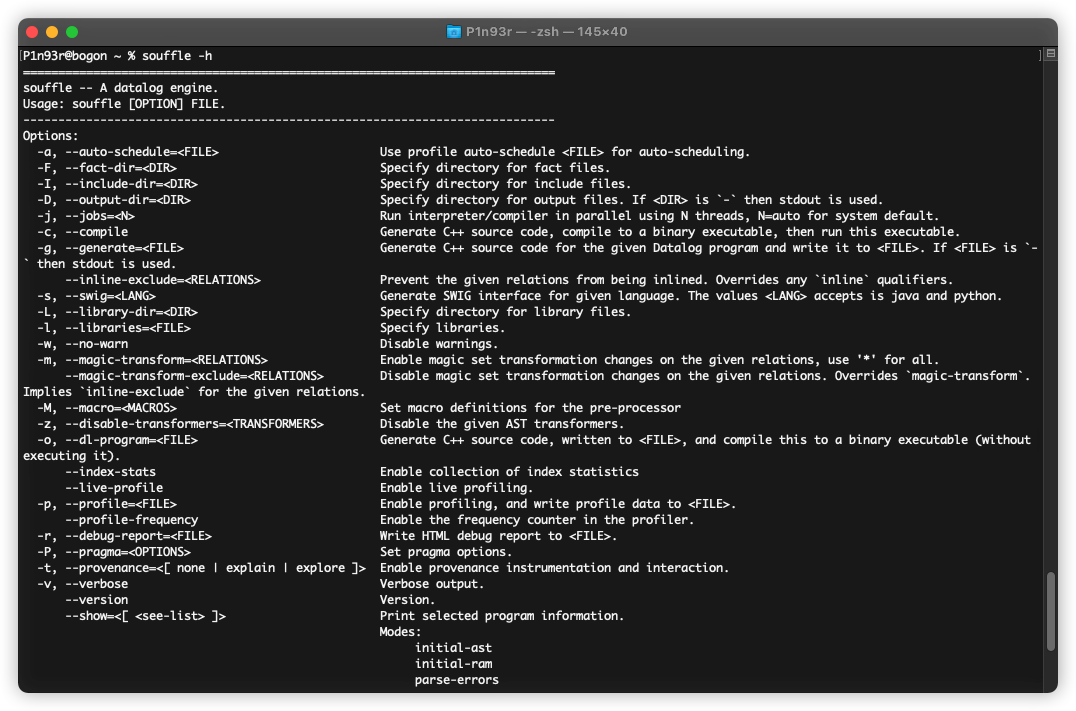# Souffle的一个简单例子

 `````` 1 2 3 4 5 6 7 8 9 10 11 12 13 `````` ``````// 声明一个Predicate edge .decl edge(x:number, y:number) // 表明需要从磁盘中读取一个edge.facts文件，这里是从文件中读取facts，也可以直接在本dl中定义facts .input edge // 声明一个Predicate path .decl path(x:number, y:number) // 表明运行结束会生成一个path.facts文件 .output path // rule推导 path(x, y) :- edge(x, y). path(x, y) :- path(x, z), edge(z, y). ``````

 ``````1 2 `````` ``````1 2 2 3 ``````

 `````` 1 2 3 4 5 6 7 8 9 10 11 12 13 14 15 16 17 18 `````` ``````P1n93r@bogon example % ll total 16 drwxr-xr-x 4 P1n93r staff 128B 4 28 19:20 . drwxr-xr-x 3 P1n93r staff 96B 4 28 19:15 .. -rw-r--r-- 1 P1n93r staff 8B 4 28 19:20 edge.facts -rw-r--r-- 1 P1n93r staff 336B 4 28 19:19 example.dl P1n93r@bogon example % **souffle -F. -D. example.dl** P1n93r@bogon example % ll total 24 drwxr-xr-x 5 P1n93r staff 160B 4 28 19:20 . drwxr-xr-x 3 P1n93r staff 96B 4 28 19:15 .. -rw-r--r-- 1 P1n93r staff 8B 4 28 19:20 edge.facts -rw-r--r-- 1 P1n93r staff 336B 4 28 19:19 example.dl -rw-r--r-- 1 P1n93r staff 12B 4 28 19:20 **path.csv** P1n93r@bogon example % cat path.csv 1 2 1 3 2 3 ``````

 ``````1 2 3 `````` ``````P1n93r@bogon example % **souffle -F. -D. -rexample.html example.dl** P1n93r@bogon example % ll example.html -rw-r--r--@ 1 P1n93r staff 76K 4 28 19:29 example.html ``````

 `````` 1 2 3 4 5 6 7 8 9 10 11 12 13 14 15 16 17 18 19 20 21 22 23 24 25 26 27 28 29 30 31 32 33 34 35 36 37 38 39 40 `````` ``````P1n93r@bogon example % souffle -F. -D. -pexample.log example.dl P1n93r@bogon example % souffleprof example.log SouffleProf runtime loadtime savetime relations rules tuples generated .000s .000s .000s 2 4 5 Slowest relations to fully evaluate ----- Relation Table ----- TOT_T NREC_T REC_T COPY_T LOAD_T SAVE_T TUPLES READS TUP/s ID NAME .000s .000s .000s .000s .000s .000s 3 1 20.0K R2 path .000s .000s .000s .000s .000s .000s 2 0 2 R1 edge Slowest rules to fully evaluate ----- Rule Table ----- TOT_T NREC_T REC_T TUPLES TUP/s ID RELATION .000s .000s .000s 1 55.5556 C2.1 path .000s .000s .000s 2 166.667 N2.1 path cpu total .009s 211% 190% * 169% * 148% * 126% * 105% * 84% ******* 63% ******* 42% ******* 21% ******* -------- > rel ----- Relation Table ----- TOT_T NREC_T REC_T COPY_T LOAD_T SAVE_T TUPLES READS TUP/s ID NAME .000s .000s .000s .000s .000s .000s 3 1 20.0K R2 path .000s .000s .000s .000s .000s .000s 2 0 2 R1 edge ``````

# First Example

## 传递闭包分析示例（Transitive clousure）

 ``````1 2 3 4 5 6 7 8 9 `````` ``````.decl edge(n: symbol, m: symbol) edge("a", "b"). /* facts of edge */ edge("b", "c"). edge("c", "b"). edge("c", "d"). .decl reachable (n: symbol, m: symbol) .output reachable // output relation reachable reachable(x, y):- edge(x, y). // base rule reachable(x, z):- edge(x, y), reachable(y, z). // inductive rule ``````

 `````` 1 2 3 4 5 6 7 8 9 10 11 12 13 14 15 16 17 `````` ``````P1n93r@bogon example % souffle -F . -D . test.dl P1n93r@bogon example % ll total 16 drwxr-xr-x 4 P1n93r staff 128B 4 29 10:36 . drwxr-xr-x 4 P1n93r staff 128B 4 28 19:33 .. -rw-r--r-- 1 P1n93r staff 36B 4 29 10:36 reachable.csv -rw-r--r-- 1 P1n93r staff 312B 4 29 10:35 test.dl P1n93r@bogon example % cat reachable.csv a b a c a d b b b c b d c b c c c d ``````

## 同代分析示例（Same generation example）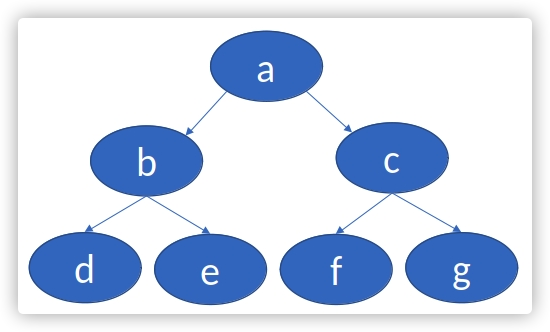`````` 1 2 3 4 5 6 7 8 9 10 11 12 13 14 15 16 17 18 19 20 21 `````` ``````// 定义一个谓词，用于描述 子-父 关系 .decl Parent(n: symbol, m: symbol) // Parent谓词的一系列事实（Facts） Parent("d", "b"). Parent("e", "b"). Parent("f","c"). Parent("g", "c"). Parent("b", "a"). Parent("c","a"). // 定义一个谓词，用于描述单个节点 .decl Node(n: symbol) Node(x) :- Parent(x, _). Node(x) :- Parent(_, x). // 定义一个谓词，用于描述同代关系 .decl SameGeneration (n: symbol, m: symbol) // 定义两条rule // 对于某个节点，自己和自己是同代 SameGeneration(x, x):- Node(x). // 如果两个子节点的父节点是同代，那么这两个子节点是同代 SameGeneration(x, y):- Parent(x,p), SameGeneration(p,q), Parent(y,q). .output SameGeneration ``````

 `````` 1 2 3 4 5 6 7 8 9 10 11 12 13 14 15 16 17 18 19 20 21 22 23 24 25 26 27 28 29 `````` ``````P1n93r@bogon example % souffle -F . -D . test.dl P1n93r@bogon example % ll total 16 drwxr-xr-x 4 P1n93r staff 128B 4 29 11:00 . drwxr-xr-x 4 P1n93r staff 128B 4 28 19:33 .. -rw-r--r-- 1 P1n93r staff 84B 4 29 11:00 SameGeneration.csv -rw-r--r-- 1 P1n93r staff 732B 4 29 11:00 test.dl P1n93r@bogon example % cat SameGeneration.csv d d d e d f d g b b b c e d e e e f e g f d f e f f f g c b c c g d g e g f g g a a ``````

## 数据流分析示例

• 如果v在p点有定值d，存在一条从p到q的路径，在这个路径上没有其他的定值点，则称v的定值d到达（reaching）p；
• 如果从p到q的路径上有其他对于v的定值，我们就说v的定值d被kill掉了；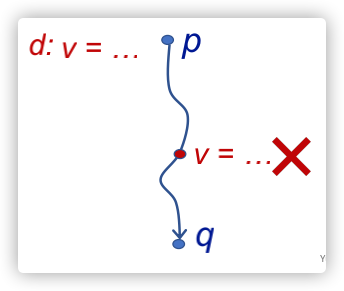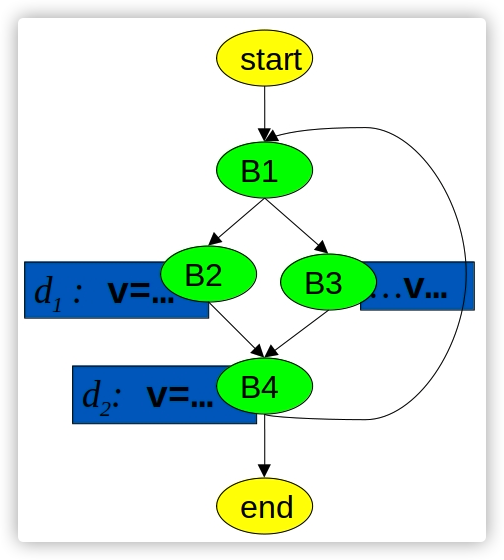`````` 1 2 3 4 5 6 7 8 9 10 11 12 13 14 15 16 17 18 19 20 21 22 23 24 25 26 27 28 29 30 31 `````` ``````// define control flow graph // via the Edge relation .decl Edge(n: symbol, m: symbol) Edge("start", "b1"). Edge("b1", "b2"). Edge("b1", "b3"). Edge("b2", "b4"). Edge("b3", "b4"). Edge("b4", "b1"). Edge("b4", "end"). // Generating Definitions .decl GenDef(n: symbol, d:symbol) GenDef("b2", "d1"). GenDef("b4", "d2"). // Killing Definitions .decl KillDef(n: symbol, d:symbol) KillDef("b4", "d1"). KillDef("b2", "d2"). // Reachable .decl Reachable(n: symbol, d:symbol) // gen点意味着当前定值一定可达gen点 Reachable(u,d) :- GenDef(u,d). // 这个地方souffle官方写的是：Reachable(v,d) :- Edge(u,v), Reachable(u,d), !KillDef(u,d). // 我认为souffle官方应该是写错了，从逻辑上来讲，定值可达u点，并且在v点不被kill掉，才能说定值可到v // 如果是souffle官方的写法，那么逻辑就是：定值可达u点，且在u点定值不被kill掉，就说明u点可达v点，感觉是不符合定值可达分析的定义的 Reachable(v,d) :- Edge(u,v), Reachable(u,d), !KillDef(v,d). .output Reachable ``````

 ``````1 2 3 4 5 6 7 `````` ``````P1n93r@bogon example % souffle -F . -D . test.dl P1n93r@bogon example % cat Reachable.csv b1 d2 b2 d1 b3 d2 b4 d2 end d2 ``````

## 一些备注

souffle支持两种注释：

 ``````1 2 `````` ``````// 这里是注释 /* 这里也是注释 */ ``````

souffle支持C预处理器（比如定义宏），例如：

 ``````1 2 `````` ``````#include "myprog.dl" #define MYPLUS(a,b) (a+b) ``````

# 关系（Relations）

## 关系声明

 ``````1 2 `````` ``````// 定义了一个关系edge，代表一系列特定元组 (a, b) 存在edge这个关系，a和b的类型都是symbol，这是一种类似strings的类型 .decl edge(a:symbol, b:symbol) ``````

## I/O指令

• `.input <relation-name>` 指令：从 `<relation-name>.facts` 中加载facts，默认使用tab进行数据分隔；
• `.out <relation-name>` 指令：默认情况下，将分析得出的facts写出到 `<relation-name>.csv` 文件中；
• `.printsize <relation-name>` 指令：在控制台中打印给定关系的facts数量；

## 语法糖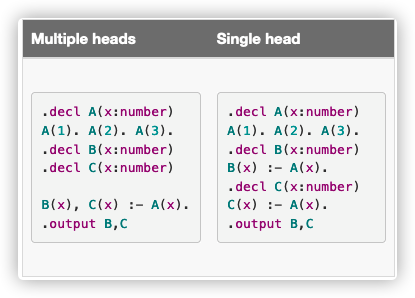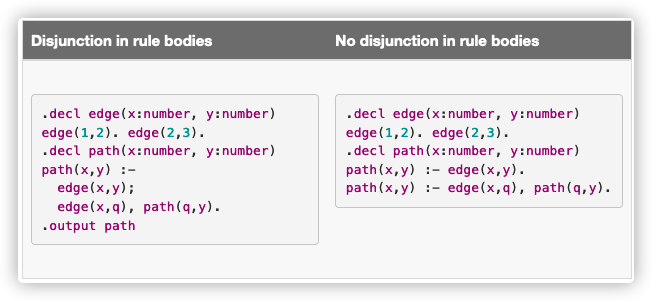# 属性的类型（Type system for attributes）

souffle的类型是静态的，必须在编译之前确定关系（relation）的属性，并在编译时进行类型检查；

## 原始类型

souffle存在两种原始类型：

• symbol：它包含所有的字符串，它的内部实现是一个ordinal number；
• number：和 int 类似；

# 算术表达式

souffle支持算术函子（arithmetic functors），扩展了传统的datalog语义；函子中使用的变量必须要能终止，也就是意味着函子中使用的变量不能是无限的，一个例子如下，使用了算术运算符 `<`

 ``````1 2 3 4 `````` ``````.decl A(n: number) .output A A(1). A(x+1) :- A(x), x < 9. ``````

souffle支持的算术函子如下：

• 加法：`x+y`
• 减法：`x-y`
• 除法：`x/y`
• 乘法：`x*y`
• 模数：`a%b`
• 幂运算：`a^b`
• 计数器：`autonic()`
• 位操作：`x band y``x bor y``x bxor y``bnot x`
• 逻辑操作：`x land y``x lor y``lnot x`

souffle支持如下算术约束：

• 小于：`a < b`
• 小于或等于：`a <= b`
• 等于：`a = b`
• 不等于：`a != b`
• 大于或等于：`a >= b`
• 大于：`a > b`

 ``````1 2 3 4 `````` ``````.decl A(x:number) A(4711). A(0b101). A(0xaffe). ``````

Notice: 在facts文件中，只支持十进制；

## 数字编码（Number encoding）

• 0代表false；
• 非0代表true；

 ``````1 2 3 `````` ``````.decl A(x:number) .output A A(0 lor 1). ``````

## autonic()函子

 ``````1 2 3 4 5 6 `````` ``````.decl A(x: symbol) A("a"). A("b"). A("c"). A("d"). .decl B(x: symbol, y: number) .output B B(x, autoinc()) :- A(x). ``````

 ``````1 2 3 4 5 6 `````` ``````P1n93r@bogon example % souffle -F . -D . test.dl P1n93r@bogon example % cat B.csv a 0 b 1 c 2 d 3 ``````

# Aggregation（聚合）

Soufflé 中的聚合（aggregation）是指使用特定的函子来汇总有关查询的信息。聚合只能应用于 Soufflé 中的稳定关系。聚合的类型包括计数、查找一组数字的最小值/最大值以及求和。通常在 Soufflé 中，信息不能从子目标（聚合函子的参数）流向外部范围。例如，如果希望找到关系 Cost(x) 的最小值，无法找到使 Cost(x) 最小化的特定 x 值。因为实际上，这样的 x 值可能不是唯一的。

## 计数（Counting）

 ``````1 2 3 4 5 6 7 8 `````` ``````.decl Car(name: symbol, colour:symbol) Car("Audi", "blue"). Car("VW", "red"). Car("BMW", "blue"). .decl BlueCarCount(x: number) BlueCarCount(c) :- c = count:{Car(_,"blue")}. .output BlueCarCount ``````

 ``````1 2 3 4 5 6 7 8 9 `````` ``````P1n93r@bogon example % souffle -F . -D . test.dl P1n93r@bogon example % ll total 16 drwxr-xr-x 4 P1n93r staff 128B 4 29 17:21 . drwxr-xr-x 4 P1n93r staff 128B 4 28 19:33 .. -rw-r--r-- 1 P1n93r staff 2B 4 29 17:21 BlueCarCount.csv -rw-r--r-- 1 P1n93r staff 196B 4 29 17:21 test.dl P1n93r@bogon example % cat BlueCarCount.csv 2 ``````

## 求最大/最小/和

 ``````1 2 3 4 5 `````` ``````.decl A(n:number) A(1). A(10). A(100). .decl MaxA(x: number) MaxA(y) :- y = max x:{A(x)}. .output MaxA ``````

• 求最小值：`min <var>:{<sub-goal(<var>)>}`
• 求和： `sum <var>:{<sub-goal(<var>)>}`

# 记录（Records）

 ``````1 `````` ``````.type = [ : , ..., : ] ``````

 `````` 1 2 3 4 5 6 7 8 9 10 11 `````` ``````// Pair of numbers .type Pair = [a:number, b:number] .decl A(p: Pair) // declare a set of pairs A([1,2]). A([3,4]). A([4,5]). .decl Flatten(a:number, b:number) .output Flatten Flatten(a,b) :- A([a,b]). ``````

## 记录的内部结构（Overview of record internals）

 ``````1 2 3 4 5 `````` ``````.type Pair = [a: number, b: number] .decl A(p: Pair) A([1,2]). A([3,4]). A([4,5]). ``````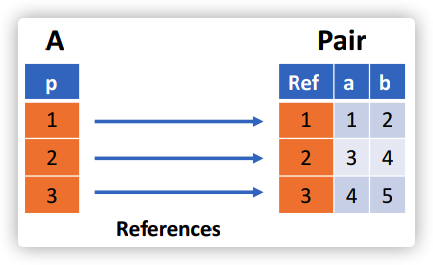## 递归记录（Recursive records）

Soufflé 中允许递归定义的记录。递归因 `nil` 记录的存在而终止。例如如下例子(souffle官方的又写错了)：

 `````` 1 2 3 4 5 6 7 8 9 10 `````` ``````.type IntList = [next: IntList, x: number] .decl L(l: IntList) L([nil,10]). // [r1,x+10] 代表需要推导的下一个IntList，其中r1代表上一个IntList，r2也是代表需要推导出的下一个IntList（独立于[r1,x+10]），这个需要推导出来的r2需要满足[r1, x],也就是next为r1（上一个IntList） L([r1,x+10]) :- L(r2), r2=[r1,x], x < 30. .decl Flatten(x: number) Flatten(x) :- L([_,x]). .output Flatten ``````

 ``````1 2 3 4 5 `````` ``````P1n93r@bogon example % souffle -F . -D . test.dl P1n93r@bogon example % cat Flatten.csv 10 20 30 ``````